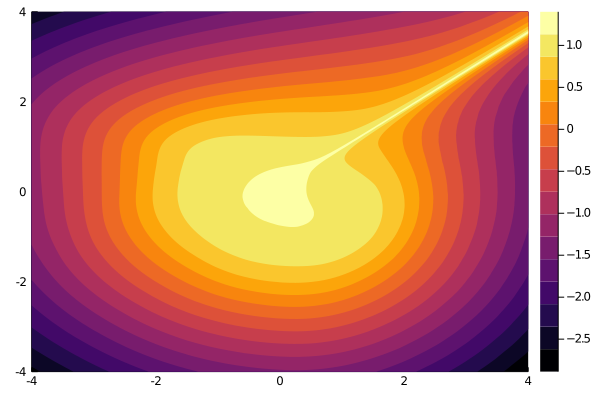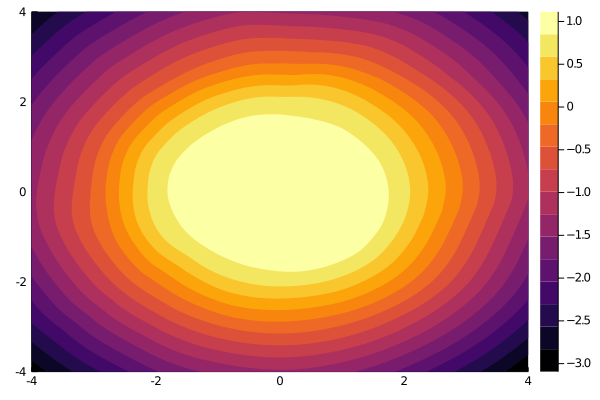# Augmented Neural Ordinary Differential Equations

## Copy-Pasteable Code

using DiffEqFlux, DifferentialEquations
using Statistics, LinearAlgebra, Plots

direction = randn(dim)
unit_direction = direction ./ norm(direction)
return distance .* unit_direction
end

num_samples_inner, num_samples_outer; batch_size = 64)
data = []
labels = []
for _ in 1:num_samples_inner
push!(labels, ones(1, 1))
end
for _ in 1:num_samples_outer
push!(labels, -ones(1, 1))
end
data = cat(data..., dims=2)
labels = cat(labels..., dims=2)
return DataLoader((data |> gpu, labels |> gpu); batchsize=batch_size, shuffle=true,
partial=false)
end

diffeqarray_to_array(x) = reshape(gpu(x), size(x)[1:2])

function construct_model(out_dim, input_dim, hidden_dim, augment_dim)
input_dim = input_dim + augment_dim
node = NeuralODE(Chain(Dense(input_dim, hidden_dim, relu),
Dense(hidden_dim, hidden_dim, relu),
Dense(hidden_dim, input_dim)) |> gpu,
(0.f0, 1.f0), Tsit5(), save_everystep = false,
reltol = 1e-3, abstol = 1e-3, save_start = false) |> gpu
node = augment_dim == 0 ? node : AugmentedNDELayer(node, augment_dim)
return Chain((x, p=node.p) -> node(x, p),
diffeqarray_to_array,
Dense(input_dim, out_dim) |> gpu), node.p |> gpu
end

function plot_contour(model, npoints = 300)
grid_points = zeros(2, npoints ^ 2)
idx = 1
x = range(-4.0, 4.0, length = npoints)
y = range(-4.0, 4.0, length = npoints)
for x1 in x, x2 in y
grid_points[:, idx] .= [x1, x2]
idx += 1
end
sol = reshape(model(grid_points |> gpu), npoints, npoints) |> cpu

return contour(x, y, sol, fill = true, linewidth=0.0)
end

loss_node(x, y) = mean((model(x) .- y) .^ 2)

println("Generating Dataset")

dataloader = concentric_sphere(2, (0.0, 2.0), (3.0, 4.0), 2000, 2000; batch_size = 256)

cb = function()
global iter += 1
if iter % 10 == 0
println("Iteration $iter || Loss =$(loss_node(dataloader.data, dataloader.data))")
end
end

model, parameters = construct_model(1, 2, 64, 0)
iter = 0

println("Training Neural ODE")

for _ in 1:10
Flux.train!(loss_node, Flux.params([parameters, model]), dataloader, opt, cb = cb)
end

plt_node = plot_contour(model)

model, parameters = construct_model(1, 2, 64, 1)
iter = 0

println()
println("Training Augmented Neural ODE")

for _ in 1:10
Flux.train!(loss_node, Flux.params([parameters, model]), dataloader, opt, cb = cb)
end

plt_anode = plot_contour(model)

# Step-by-Step Explanation

using DiffEqFlux, DifferentialEquations
using Statistics, LinearAlgebra, Plots
import Flux.Data: DataLoader

## Generating a toy dataset

In this example, we will be using data sampled uniformly in two concentric circles and then train our Neural ODEs to do regression on that values. We assign 1 to any point which lies inside the inner circle, and -1 to any point which lies between the inner and outer circle. Our first function random_point_in_sphere samples points uniformly between 2 concentric circles/spheres of radii min_radius and max_radius respectively.

function random_point_in_sphere(dim, min_radius, max_radius)
direction = randn(dim)
unit_direction = direction ./ norm(direction)
return distance .* unit_direction
end

Next, we will construct a dataset of these points and use Flux's DataLoader to automatically minibatch and shuffle the data.

function concentric_sphere(dim, inner_radius_range, outer_radius_range,
num_samples_inner, num_samples_outer; batch_size = 64)
data = []
labels = []
for _ in 1:num_samples_inner
push!(labels, ones(1, 1))
end
for _ in 1:num_samples_outer
push!(labels, -ones(1, 1))
end
data = cat(data..., dims=2)
labels = cat(labels..., dims=2)
return DataLoader(data |> gpu, labels |> gpu; batchsize=batch_size, shuffle=true,
partial=false)
end

## Models

We consider 2 models in this tutorial. The first is a simple Neural ODE which is described in detail in this tutorial. The other one is an Augmented Neural ODE . The idea behind this layer is very simple. It augments the input to the Neural DE Layer by appending zeros. So in order to use any arbitrary DE Layer in combination with this layer, simply assume that the input to the DE Layer is of size size(x, 1) + augment_dim instead of size(x, 1) and construct that layer accordingly.

In order to run the models on GPU, we need to manually transfer the models to GPU. First one is the network predicting the derivatives inside the Neural ODE and the other one is the last layer in the Chain.

diffeqarray_to_array(x) = reshape(gpu(x), size(x)[1:2])

function construct_model(out_dim, input_dim, hidden_dim, augment_dim)
input_dim = input_dim + augment_dim
node = NeuralODE(Chain(Dense(input_dim, hidden_dim, relu),
Dense(hidden_dim, hidden_dim, relu),
Dense(hidden_dim, input_dim)) |> gpu,
(0.f0, 1.f0), Tsit5(), save_everystep = false,
reltol = 1e-3, abstol = 1e-3, save_start = false) |> gpu
node = augment_dim == 0 ? node : (AugmentedNDELayer(node, augment_dim) |> gpu)
return Chain((x, p=node.p) -> node(x, p),
diffeqarray_to_array,
Dense(input_dim, out_dim) |> gpu), node.p |> gpu
end

## Plotting the Results

Here, we define an utility to plot our model regression results as a heatmap.

function plot_contour(model, npoints = 300)
grid_points = zeros(2, npoints ^ 2)
idx = 1
x = range(-4.0, 4.0, length = npoints)
y = range(-4.0, 4.0, length = npoints)
for x1 in x, x2 in y
grid_points[:, idx] .= [x1, x2]
idx += 1
end
sol = reshape(model(grid_points |> gpu), npoints, npoints) |> cpu

return contour(x, y, sol, fill = true, linewidth=0.0)
end

## Training Parameters

### Loss Functions

We use the L2 distance between the model prediction model(x) and the actual prediction y as the optimization objective.

loss_node(x, y) = mean((model(x) .- y) .^ 2)

### Dataset

Next, we generate the dataset. We restrict ourselves to 2 dimensions as it is easy to visualize. We sample a total of 4000 data points.

dataloader = concentric_sphere(2, (0.0, 2.0), (3.0, 4.0), 2000, 2000; batch_size = 256)

### Callback Function

Additionally we define a callback function which displays the total loss at specific intervals.

cb = function()
global iter += 1
if iter % 10 == 1
println("Iteration $iter || Loss =$(loss_node(dataloader.data, dataloader.data))")
end
end

### Optimizer

We use ADAM as the optimizer with a learning rate of 0.005

opt = ADAM(0.005)

## Training the Neural ODE

To train our neural ode model, we need to pass the appropriate learnable parameters, parameters which is returned by the construct_models function. It is simply the node.p vector. We then train our model for 20 epochs.

model, parameters = construct_model(1, 2, 64, 0)

for _ in 1:10
Flux.train!(loss_node, Flux.params([model, parameters]), dataloader, opt, cb = cb)
end

Here is what the contour plot should look for Neural ODE. Notice that the regression is not perfect due to the thin artifact which connects the circles.## Training the Augmented Neural ODE

Our training configuration will be same as that of Neural ODE. Only in this case we have augmented the input with a single zero. This makes the problem 3 dimensional and as such it is possible to find a function which can be expressed by the neural ode. For more details and proofs please refer to .

model, parameters = construct_model(1, 2, 64, 1)

for _ in 1:10
Flux.train!(loss_node, Flux.params([model, parameters]), dataloader, opt, cb = cb)
end

For the augmented Neural ODE we notice that the artifact is gone.# Expected Output

Generating Dataset
Training Neural ODE
Iteration 10 || Loss = 0.9802582
Iteration 20 || Loss = 0.6727416
Iteration 30 || Loss = 0.5862373
Iteration 40 || Loss = 0.5278132
Iteration 50 || Loss = 0.4867624
Iteration 60 || Loss = 0.41630346
Iteration 70 || Loss = 0.3325938
Iteration 80 || Loss = 0.28235924
Iteration 90 || Loss = 0.24069068
Iteration 100 || Loss = 0.20503852
Iteration 110 || Loss = 0.17608969
Iteration 120 || Loss = 0.1491399
Iteration 130 || Loss = 0.12711425
Iteration 140 || Loss = 0.10686825
Iteration 150 || Loss = 0.089558244

Training Augmented Neural ODE
Iteration 10 || Loss = 1.3911372
Iteration 20 || Loss = 0.7694144
Iteration 30 || Loss = 0.5639633
Iteration 40 || Loss = 0.33187616
Iteration 50 || Loss = 0.14787851
Iteration 60 || Loss = 0.094676435
Iteration 70 || Loss = 0.07363529
Iteration 80 || Loss = 0.060333826
Iteration 90 || Loss = 0.04998395
Iteration 100 || Loss = 0.044843454
Iteration 110 || Loss = 0.042587914
Iteration 120 || Loss = 0.042706195
Iteration 130 || Loss = 0.040252227
Iteration 140 || Loss = 0.037686247
Iteration 150 || Loss = 0.036247417

 Dupont, Emilien, Arnaud Doucet, and Yee Whye Teh. "Augmented neural ODEs." In Proceedings of the 33rd International Conference on Neural Information Processing Systems, pp. 3140-3150. 2019.